# Graphics and Bit Operations Problems

The following problems test your knowledge of basic graphics and bit operations.

### Eighth of a Circle

 Important Write a function that draws the upper eighth of a circle centered at (0, 0) with a given radius, where the upper eighth is defined as the portion starting at 12 and going to 1:30 on a clock face. Use the following prototype: ` void drawEighthOfCircle( int radius );` The coordinate system and an example of what you are to draw are shown in Figure 11-1. You will use a function with the following prototype to draw pixels: ` void setPixel( int xCoord, int yCoord );`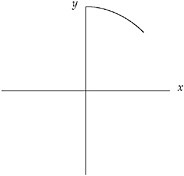Figure 11-1

This problem is not as contrived as it seems. If you were trying to implement a full-circle drawing routine, you would want to do as little calculation as possible to maintain optimum performance. Given the pixels for one-eighth of a circle, you can easily determine the pixels for the remainder of the circle from symmetry.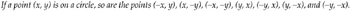This problem is an example of a scan conversion, converting a geometric drawing to a pixel-based raster image. You will need an equation for a circle before you can calculate anything. The common mathematical function that produces a circle is as follows: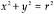The definition is nice because it contains x’s, y’s, and r’s, just like your problem and your coordinate system. You have to figure out how to determine pairs of coordinates (x, y) on the circle using the equation, x2 + y2 = r2. The easiest way to find a pair of coordinates is to set a value for one and then calculate the other. It’s more difficult to set y and calculate x because after the scan conversion there will be several x values for certain y values. Therefore, you should set x and calculate y. Doing some algebra, you can calculate y with the following equation: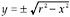In this problem you are dealing with only positive values of y, so you can ignore the negative root. This produces the following: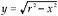For example, given an x coordinate of 3 and a radius of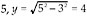. You now know how to calculate y, given x. Next, you need to determine the range of x values. x clearly starts at 0, but where does it end? Look again at the figure and try to figure out how you visually know that you are at the end of the one-eighth of the circle. In visual terms, this happens when you are farther out then you are up. In mathematical terms, this means that the x value becomes greater than the y value. Thus, you can use the x range from 0 until x > y. If you put these pieces together, you have an algorithm for drawing a circle. In outline form, it appears as follows:

` Start with x = 0 and y = r. While (y > x)     Determine the y coordinate using the equation: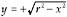Set the pixel (x, y)     Increment x `

This algorithm looks correct, but there is a subtle bug in it. The problem arises from treating the y coordinate as an integer, when often y will be a decimal value. For example, if y had the value 9.99, setPixel would truncate it to 9, rather than round to the y pixel of 10 as you probably want. One way to solve this problem is to round all values to the nearest whole integer by adding 0.5 to the y value before calling setPixel.

This change results in a much better-looking circle. The code for this algorithm is as follows:

` void drawEighthOfCircle(int radius ){     int x, y;     x = 0;     y = radius;     while( y <= x ){         y = Math.sqrt((radius * radius) – (x * x)) + 0.5;         setPixel( x, y );         x++;     } }`

What’s the efficiency of this algorithm? Its running time is O(n), where n is the number of pixels that you need to set. This is the best possible running time because any algorithm would have to call setPixel at least n times to draw the circle correctly. The function also uses the sqrt function and multiplies during each iteration of the while loop. The sqrt function and the multiplications are likely to be slow operations. Therefore, this function probably isn’t practical for most graphical applications where speed is critical. There are faster circle-drawing algorithms that don’t make repeated calls to slow functions like sqrt or have repeated multiplications, but you wouldn’t be expected to implement them during an interview.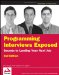Programming Interviews Exposed: Secrets to Landing Your Next Job, 2nd Edition (Programmer to Programmer)
ISBN: 047012167X
EAN: 2147483647
Year: 2007
Pages: 94

Similar book on Amazon

flylib.com © 2008-2017.
If you may any questions please contact us: flylib@qtcs.net